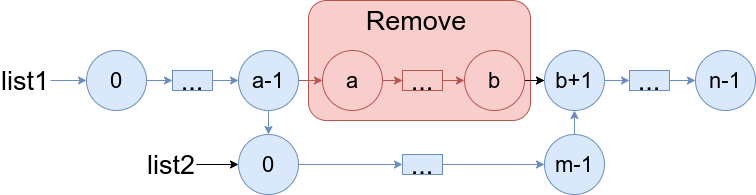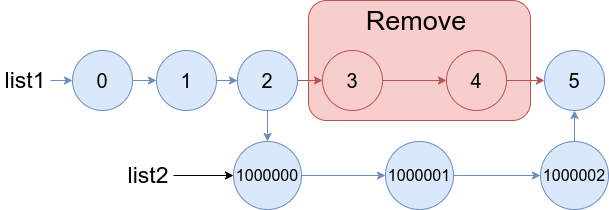Formatted question description: https://leetcode.ca/all/1669.html

# Question

You are given two linked lists: list1 and list2 of sizes n and m respectively. Remove list1’s nodes from the ath node to the bth node, and put list2 in their place. The blue edges and nodes in the following figure incidate the result:Build the result list and return its head.

Example 1:Input: list1 = [0,1,2,3,4,5], a = 3, b = 4, list2 = [1000000,1000001,1000002] Output: [0,1,2,1000000,1000001,1000002,5] Explanation: We remove the nodes 3 and 4 and put the entire list2 in their place. The blue edges and nodes in the above figure indicate the result.

Example 2:Input: list1 = [0,1,2,3,4,5,6], a = 2, b = 5, list2 = [1000000,1000001,1000002,1000003,1000004] Output: [0,1,1000000,1000001,1000002,1000003,1000004,6] Explanation: The blue edges and nodes in the above figure indicate the result.

# Code

Java

Java

/**
* public class ListNode {
*     int val;
*     ListNode next;
*     ListNode() {}
*     ListNode(int val) { this.val = val; }
*     ListNode(int val, ListNode next) { this.val = val; this.next = next; }
* }
*/
class Solution {
public ListNode mergeInBetween(ListNode list1, int a, int b, ListNode list2) {
ListNode start2 = list2, end2 = list2;
while (end2.next != null)
end2 = end2.next;
ListNode insertStart = list1, insertEnd = list1;
for (int i = 1; i < a; i++)
insertStart = insertStart.next;
for (int i = 0; i <= b; i++)
insertEnd = insertEnd.next;
insertStart.next = start2;
end2.next = insertEnd;
return list1;
}
}